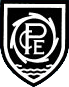# Learning  »  Class Pages  »  Year 5  »  Class Work

Maths Week 6 - Autumn 1

This week we will continue our work on calculating area, but looking at more problem solving and real life situations where we might need to know the area of a shape. We will start to look at compound shapes and how we calculate the area of these.
Maths Week 5 - Autumn 1

This week we will be moving away from perimeter and onto calculating area. We will be starting by looking at what the area of a shape is and how we can use different methods to calculate the area of basic rectangles. Some of these will include using estimates to calculate the area when a rectangle has a side with a decimal.
Maths Week 4 Autumn 1

Perimeter of rectilinear shapes

Maths Week 4 - Autumn 1
This week we will be continuing our work on finding the perimeter of rectlinear shapes. We will look at different strategies to try and find the missing sides, indluing using the bar model. The attached sheets shows some of the problems that the year 5's are looking at solving.
Maths. Week 3. Ordering numbers

The 2 documents have different levels of difficulty so that pupils can choose the right challenge. Answers are on the last page of each document.

Maths. Week 3. Autumn 1.
This week we will continue to focus on number and place value. Again, we will look at ordering numbers and we will make sure that we can EXPLAIN what we know about the numbers we are working with and also represent numbers with visual images. We have been using both Year 4 and Year 5 resources to help us remember what we learned last year and also to move our learning forwards - see the documents section above for examples of what we've been doing.

Continuing from last week, we will start to look at what a rectilinear shape is and how we calculate its perimeter. We will look at how we can avoid making simple mistakes and also how to find missing sides of the shape.
Maths. Week 2. Autumn 1.
This week we have spent time more time revising what we were learning about in Year 4 before the lockdown. We have concentrated on ordering and comparing numbers (to 100, 1000, 10,000 and 100,000). We have worked at our own pace and are making sure we REALLY understand  the value of each digit in each number.

We will also be looking at what the perimeter of a 2d shape is and how we calculate this. We will concentrate mainly on rectangles and sqaures, including what to do with missing sides. This will prepare us for next weeks work on composite rectilinear shapes.
Maths. Week 1. Autumn 1.
This week we have spent time revising what we were learning about in Year 4 before the lockdown.Some of us have rememebered lots - others need a bit more time getting our Maths brains working! So we have played lots of class games and covered areas such as number and place value (working up to 99,000 but  focusing mainly on up to 10,000) , simple number bonds and also times tables.

Pool-in-Wharfedale CE Primary School Arthington Lane, Pool-in-Wharfedale, Otley, LS21 1LG